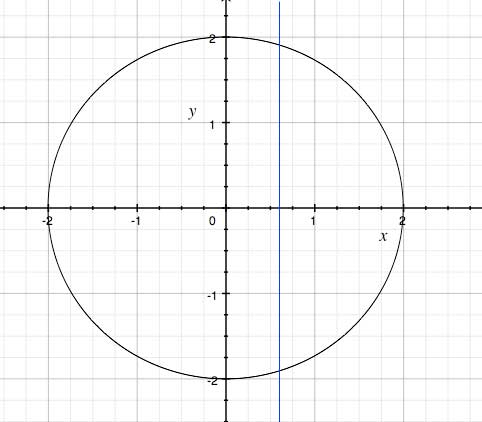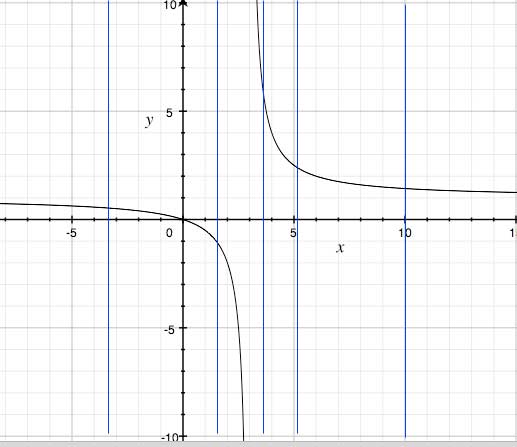SEARCH HOMEMath Central Quandaries & QueriesKatie, a student: Determine whether y is a function of x: [(x2)y]-(x2)+(4y)=0 ....thxHi Katie,

First step in determining if y is a function of x or not, is isolating y. For example, say you are given x2+y2=4

y2=4-x2

y=±√4-x2

Next step is graphing. Draw a series of vertical lines. If any of these vertical lines goes through the graph more than once, it is not a function. If each vertical line goes through the graph only once, it is a function.Clearly the vertical line goes through the graph more than once so this is not a function.

On the other hand, say you are given xy-3y-x=0

xy-3y=x

y(x-3)=x

y=x/(x-3)Looking at the graph, the vertical line crosses the graph only one time so y is a function of x. The definition of a function is for every value of x there is only one y value, that is why the vertical line test works. This graph in particular is a rational function because of the fraction.

Hope this helps,

JaniceMath Central is supported by the University of Regina and The Pacific Institute for the Mathematical Sciences.